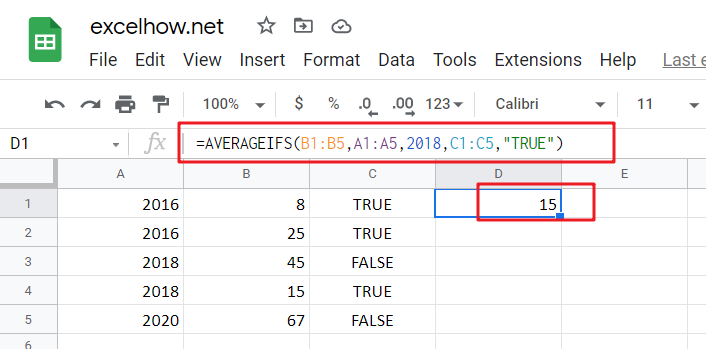# ExcelHow

This post will guide you how to use Google Sheets AVERAGEIFS function with syntax and examples in Google Spreadsheet.

## Description

The Google Sheets AVERAGEAIFS function returns the average of all numbers in a range of cells that meet multiple criteria.

The AVERAGEIFS function is a build-in function in Google Sheets and it is categorized as a Statistical Function.

## Syntax

The syntax of the AVERAGEIFS function is as below:

`= AVERAGEIFS (average_range, criteria_range1, criteria1, [criteria_range2, criteria2],…)`

Where the AVERAGEIFS function arguments are:

• Average_range – This is a required argument. The range of cells that you want to average.
• Criteria_range1  – This is a required argument. The range to apply the associated criteria.
• Criteria – This is an optional argument. The criteria used to define which cells are averaged.

### Google Sheets AVERAGEIFS function Example

The below examples will show you how to use Google Sheets AVERAGEIFS Function to return the average of all numbers in a range of cells based on multiple criteria.

#1 To get the average of all numbers in average range B1:B5 and meet the criteria that equal to “2015” in range A1:A5, and equal to “TRUE”in range C1:C5 , just using the following Google Sheets formula:

`=AVERAGEIFS(B1:B5,A1:A5,2018,C1:C5,"TRUE")`